Function Repository Resource:

# FunctionCusps

Find the cusps of a curve

Contributed by: Wolfram|Alpha Math Team
 ResourceFunction["FunctionCusps"][c,x] returns the cusps of the curve c viewed as a function of x.

## Details and Options

A cusp, or spinode, is a point where two branchs of a curve meet and the tangents of each branch are equal.

## Examples

### Basic Examples (1)

Compute the cusps of a curve:

 In:=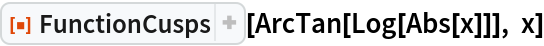Out=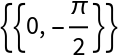### Possible Issues (2)

Note that when using fractional powers to define the expression, Surd may be necessary to extend the real-valued domain of the curve:

 In:=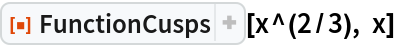Out=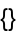In:=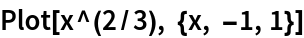Out=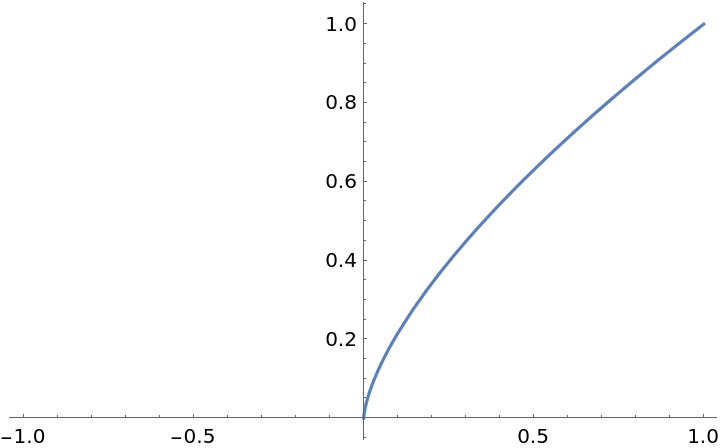In comparison with:

 In:=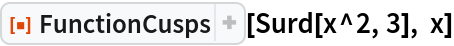Out=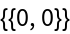In:=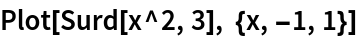Out=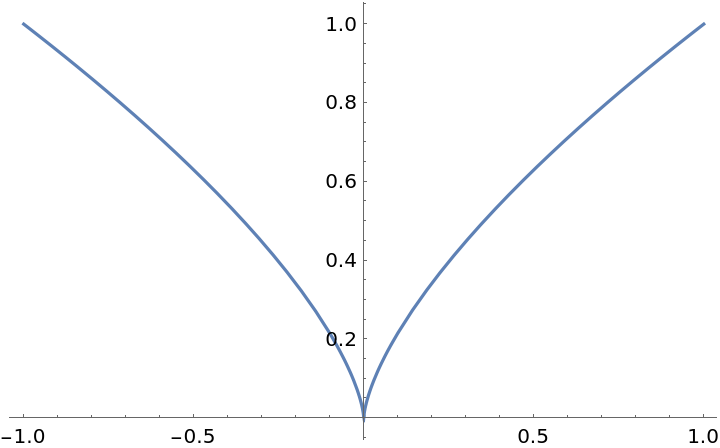Note the distinction between cusps and corners, which can be computed using the resource function FunctionCorners:

 In:=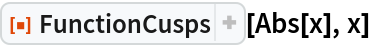Out=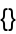In:=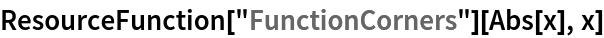Out=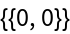## Publisher

Wolfram|Alpha Math Team

## Version History

• 3.0.0 – 23 March 2023
• 2.0.0 – 08 April 2020
• 1.0.0 – 06 April 2020